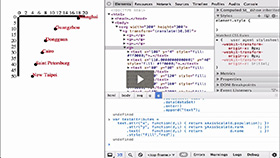Putting It All Together
Duration: 11:54
Part of Course: Introductory D3 Course
This Lesson is for subscribersUnlock this lesson NOW!
• Get expert advice on Putting It All Together
• Start mastering D3.js right now!

Takeaways:

• Then figure out what attributes of the data you are going to visualize
• Then choose what type of visualization you are going to make
• Then choose the dimensions of where you will make the visualization
• Then create the scales for the visualization, in this case - the X and Y axis scales
• Then define the Axes for the X and Y axis
• Then decide on the data representation, in this case - a circle will represent data elements
• Then define attributes for the visual data representation